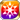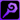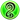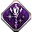# Windblown Sands of TimeIf there can be a moment when a gentle wind can be said to fulfill one's heart, then this peaceful time is surely one such occasion. "I made a hair ornament the other day, following the instructions you gave me!" The blood of the Sephira flows through her veins, making her very important to one such as I, who is responsible for the welfare of the people of the desert. Yet apart from all that, what I truly enjoy is the feel of the pleasant breeze that seems to emanate towards me by way of her presence as she gazes upon her destiny. (Notes by Nefertiti, "The Chastisement Diaries")

### Windblown Sands of Time

Raises HP by 60%/MATK Power by 20%/MATK by 20%/Strong vs Gluttony by 20%/Strong vs Wrath by 20%/Strong vs Lust by 20% for the Memento group "Desert Dwellers"

Target Buff (SelfSide) (CardLsSkill)
Stats
TypeMinMax
HP+60%+60%
Magic ATK Power+20+20
MATK+20%+20%
Strong vs <暴食>+20+20
Strong vs <憤怒>+20+20
Strong vs <色欲>+20+20

## Stats

#### Simple stats

TypeLvl 1Lvl 30Lvl 40
MDEF+10+24+30

LevelMDEF
1+10
2+10
3+11
4+11
5+12
6+12
7+13
8+13
9+14
10+14
LevelMDEF
11+15
12+15
13+16
14+16
15+17
16+17
17+18
18+18
19+19
20+19
LevelMDEF
21+20
22+20
23+21
24+21
25+22
26+22
27+23
28+23
29+24
30+24
LevelMDEF
31+25
32+25
33+26
34+26
35+27
36+27
37+28
38+28
39+29
40+30

## Group skills

### Desert Zone's Goring Strike

#### Simple stats

TypeLvl 1Lvl 30Lvl 40
Pierce ATK Power+3+8+10

#### Detailed stats

LevelPierce ATK Power
1+3
2+3
3+3
4+3
5+3
6+3
7+4
8+4
9+4
10+4
LevelPierce ATK Power
11+4
12+4
13+5
14+5
15+5
16+5
17+5
18+6
19+6
20+6
LevelPierce ATK Power
21+6
22+6
23+6
24+7
25+7
26+7
27+7
28+7
29+8
30+8
LevelPierce ATK Power
31+8
32+8
33+8
34+8
35+9
36+9
37+9
38+9
39+9
40+10

#### Limit Break

TypeLB1LB2LB3LB4LB5
Pierce ATK Power+1+2+3+4+6

### Desert Zone's Goring Strike

#### Simple stats

TypeLvl 1Lvl 30Lvl 40
Pierce ATK Power+3+8+10

#### Detailed stats

LevelPierce ATK Power
1+3
2+3
3+3
4+3
5+3
6+3
7+4
8+4
9+4
10+4
LevelPierce ATK Power
11+4
12+4
13+5
14+5
15+5
16+5
17+5
18+6
19+6
20+6
LevelPierce ATK Power
21+6
22+6
23+6
24+7
25+7
26+7
27+7
28+7
29+8
30+8
LevelPierce ATK Power
31+8
32+8
33+8
34+8
35+9
36+9
37+9
38+9
39+9
40+10

#### Limit Break

TypeLB1LB2LB3LB4LB5
Pierce ATK Power+1+2+3+4+6

### Golden Sands

#### Simple stats

TypeLvl 1Lvl 30Lvl 40
MATK+20+49+60
JUMP+1+1+1

LevelMATKJUMP
1+20+1
2+21+1
3+22+1
4+23+1
5+24+1
6+25+1
7+26+1
8+27+1
9+28+1
10+29+1
LevelMATKJUMP
11+30+1
12+31+1
13+32+1
14+33+1
15+34+1
16+35+1
17+36+1
18+37+1
19+38+1
20+39+1
LevelMATKJUMP
21+40+1
22+41+1
23+42+1
24+43+1
25+44+1
26+45+1
27+46+1
28+47+1
29+48+1
30+49+1
LevelMATKJUMP
31+50+1
32+51+1
33+52+1
34+53+1
35+54+1
36+55+1
37+56+1
38+57+1
39+58+1
40+60+1

#### Limit Break

TypeLB1LB2LB3LB4LB5
MATK+6+13+21+28+36

### On Beautiful Flowers

#### Simple stats

TypeLvl 1Lvl 30Lvl 40
HP+100+248+300
LUCK+20+49+60
Magic ATK Power+3+8+10

#### Detailed stats

LevelHPLUCKMagic ATK Power
1+100+20+3
2+105+21+3
3+110+22+3
4+115+23+3
5+120+24+3
6+125+25+3
7+130+26+4
8+135+27+4
9+141+28+4
10+146+29+4
LevelHPLUCKMagic ATK Power
11+151+30+4
12+156+31+4
13+161+32+5
14+166+33+5
15+171+34+5
16+176+35+5
17+182+36+5
18+187+37+6
19+192+38+6
20+197+39+6
LevelHPLUCKMagic ATK Power
21+202+40+6
22+207+41+6
23+212+42+6
24+217+43+7
25+223+44+7
26+228+45+7
27+233+46+7
28+238+47+7
29+243+48+8
30+248+49+8
LevelHPLUCKMagic ATK Power
31+253+50+8
32+258+51+8
33+264+52+8
34+269+53+8
35+274+54+9
36+279+55+9
37+284+56+9
38+289+57+9
39+294+58+9
40+300+60+10

#### Limit Break

TypeLB1LB2LB3LB4LB5
HP+36+72+108+144+180
LUCK+7+14+21+28+36
Magic ATK Power+1+2+3+4+6

TypeLB5
Buff Duration+1

## Vision abilities

### Limit Reached Bonus

#### Ability base

• Slot:Support

Revealed after Limit Reached. Raises AGI after appearing on map.

#### Ability max

• Slot:Action

Raises AGI after appearing on map.

### Sands' End Waltz

#### Ability base

• Slot:Action
• DMG Type:Magical
• ATK Type:Magic
• Element:Wind

Wind Mag ATK on units within area that increases in power according to own MATK/LUCK & lowers Magic Res for three turns [Range: 3, Area: Square (3), Height Range: 2]

Skill Effect
TypeMinMax
Attack160%225%
• Charges: 2
• Jewel Cost: 50
• Height: 2
• Select Range: Diamond
• Range: 0-3
• Select Scope: Square
• Scope: 1

#### Ability max

• Slot:Action
• DMG Type:Magical
• ATK Type:Magic
• Element:Wind

Wind Mag attack on units within area that increases in power according to own MATK/LUCK & lowers Magic Res for three turns [Range: 3, Area: Square (3), Height Range: 2]

Skill Effect
TypeMinMax
Attack300%300%
• Charges: 2
• Jewel Cost: 50
• Height: 2
• Select Range: Diamond
• Range: 0-3
• Select Scope: Square
• Scope: 1

### Sands' End Waltz

#### Ability base

• Slot:Action
• DMG Type:Magical
• ATK Type:Magic
• Element:Wind

Wind Mag ATK on units within area that increases in power according to own MATK/LUCK & lowers Magic Res for three turns [Range: 3, Area: Square (3), Height Range: 2]

Skill Effect
TypeMinMax
Attack160%225%
• Charges: 2
• Jewel Cost: 50
• Height: 2
• Select Range: Diamond
• Range: 0-3
• Select Scope: Square
• Scope: 1

#### Ability max

• Slot:Action
• DMG Type:Magical
• ATK Type:Magic
• Element:Wind

Wind Mag attack on units within area that increases in power according to own MATK/LUCK & lowers Magic Res for three turns [Range: 3, Area: Square (3), Height Range: 2]

Skill Effect
TypeMinMax
Attack300%300%
• Charges: 2
• Jewel Cost: 50
• Height: 2
• Select Range: Diamond
• Range: 0-3
• Select Scope: Square
• Scope: 1

#### Job restrictionSage[[Category:Strong vs <暴食>]][[Category:Strong vs <憤怒>]][[Category:Strong vs <色欲>]]